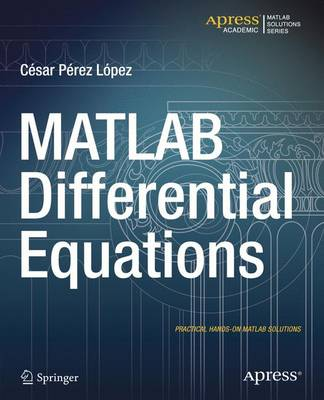•# MATLAB Differential Equations (Paperback)

(author)
£32.99
Paperback 188 Pages / Published: 11/09/2014
• We can order this

Usually dispatched within 3 weeks

• This item has been added to your basket

MATLAB is a high-level language and environment for numerical computation, visualization, and programming. Using MATLAB, you can analyze data, develop algorithms, and create models and applications. The language, tools, and built-in math functions enable you to explore multiple approaches and reach a solution faster than with spreadsheets or traditional programming languages, such as C/C++ or Java.

MATLAB Differential Equations introduces you to the MATLAB language with practical hands-on instructions and results, allowing you to quickly achieve your goals. In addition to giving an introduction to the MATLAB environment and MATLAB programming, this book provides all the material needed to work on differential equations using MATLAB. It includes techniques for solving ordinary and partial differential equations of various kinds, and systems of such equations, either symbolically or using numerical methods (Euler's method, Heun's method, the Taylor series method, the Runge-Kutta method,...). It also describes how to implement mathematical tools such as the Laplace transform, orthogonal polynomials, and special functions (Airy and Bessel functions), and find solutions of finite difference equations.

Publisher: APress
ISBN: 9781484203118
Number of pages: 188
Weight: 3614 g
Dimensions: 235 x 191 x 10 mm
Edition: 1st ed.

## Reviews

Sign In To Write A Review

Please sign in to write a review

Your review has been submitted successfully.